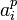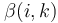# Power-commutator presentation

A power-commutator presentation of a group$G$ for a prime number$p$ is a presentation with generating set$a_i, i \in I$ for a totally ordered indexing set$I$ and relations of the form:
• power relations:$a_i^p$ is written as a product of powers of$a_k, k > i$, with the$k$s in increasing order as we go from left to right in the product. The exponent on$a_k$ for$k > i$ is denoted$\beta(i,k)$.
• commutator relations: The commutator$[a_i,a_j]$ is written as a product of powers of$a_k, k > \max \{ i , j \}$, with the$k$s in increasing order as we go from left to right in the product. The exponent of$a_k$ for$k > \max \{ i , j \}$ is denoted$\beta(i,j,k)$.
For a group of prime power order$p^n$, a power-commutator presentation is termed consistent if it uses exactly$n$ generators.